# Surface tension

The surface tension,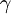$\gamma$, is a measure of the work required to create an interface between two bulk phases.

## Thermodynamics

In the Canonical ensemble the surface tension is formally given as: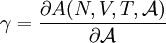$\gamma = \frac{ \partial A (N,V,T, {\mathcal A} )}{\partial {\mathcal A} }$;

where

•$A$ is the Helmholtz energy function
•$N$ is the number of particles
•$V$ is the volume
•$T$ is the temperature
•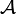${\mathcal A}$ is the surface area

## Computer Simulation

Different techniques may be used to compute this quantity, such as the traditional stress tensor route or the more recent test area method. A review can be found in the paper by Gloor et al. (Ref. 1).

## Liquid-Vapour Interfaces of one component systems

### Binder procedure

Here, only an outline of the procedure is presented, more details can be found in Reference 2. For given conditions of volume and temperature, the Helmholtz energy function is computed as a function of the number of molecules,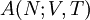$A(N;V,T)$. The calculation is usually carried out using Monte Carlo simulation using periodic boundary conditions If liquid-vapour equilibrium occurs, a plot of the chemical potential,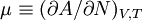$\mu \equiv (\partial A/\partial N)_{V,T}$, as a function of$N$ shows a loop. Using basic thermodynamic procedures (Maxwell's equal area construction) it is possible to compute the densities of the two phases;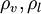$\rho_v, \rho_l$ at liquid-vapour equilibrium. Considering the thermodynamic limit for densities$\rho$ with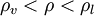$\rho_v < \rho < \rho_l$ the Helmholtz energy function will be: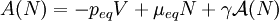$A(N) = - p_{eq} V + \mu_{eq} N + \gamma {\mathcal A}(N)$

where the quantities with the subindex "eq" are those corresponding to the fluid-phase equilibrium situation. From the previous equation one can write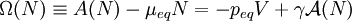$\Omega (N) \equiv A(N) - \mu_{eq} N = - p_{eq} V + \gamma {\mathcal A}(N)$.

For appropriate values of$N$ one can estimate the value of the surface area,${\mathcal A}$ (See MacDowell et al., Ref. 3), and compute$\gamma$ directly as: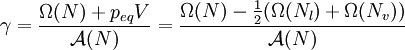$\gamma = \frac{ \Omega(N) + p_{eq} V } { {\mathcal A}(N) } = \frac{ \Omega(N) - \frac{1}{2}(\Omega(N_l)+\Omega(N_v)) }{{\mathcal A}(N)}$

where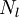$N_l$ and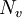$N_v$ are given by: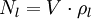$N_l = V \cdot \rho_l$ and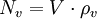$N_v = V \cdot \rho_v$

### Explicit interfaces

In these methods one performs a direct simulation of the two-phase system. Periodic boundary conditions are usually employed. Simulation boxes are elongated in one direction, and the interfaces are built (and expected to stay) perpendicular to such a direction. Taking into account the canonical ensemble definition (see above), one computes the change in the Helmholtz energy function when a small (differential) change of the surface area is performed at constant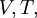$V, T,$ and$N$. The explicit equations can be written in terms of the diagonal components of the pressure tensor of the system. Mechanical arguments can also be invoked to arrive at equivalent conclusions (see Ref 1 for a detailed discussion of these issues).

### System-size analysis

The system-size dependence of the results for$\gamma$ have to be taken into account in order to obtain accurate results for model systems. Spurious effects that occur due to small system sizes can appear in the explicit interface methods. (See P.Orea et al. Ref. 4).

## Mixtures

Different ensembles can be used to compute the surface tension between two phases in the case of mixtures (See for example see Y. Zhang et al. Ref 5). The simulation techniques are essentially the same as those for one-component systems, but different ensembles can be more adequate. For instance, for binary mixtures (with components 1 and 2), the isothermal-isobaric ensemble,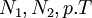$N_1,N_2,p.T$ is a more natural ensemble to compute$\gamma$ using explicit interface techniques (See Ref. 6 as an example). In the case of the Binder technique the analysis can be carried out by fixing the total number of particles: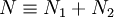$N \equiv N_1 + N_2$, the pressure,$p$, and the temperature$T$. Then one will have to compute the variation of the adequate thermodynamic potential as a function of the composition, e.g.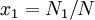$x_1 = N_1/N$.